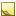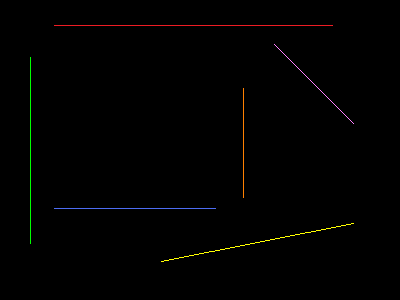﻿ HoughLine Class# HoughLine Class

Hough line.Inheritance Hierarchy
SystemObject
Accord.ImagingHoughLine

Namespace:  Accord.Imaging
Assembly:  Accord.Imaging (in Accord.Imaging.dll) Version: 3.8.0Syntax
`public class HoughLine : IComparable<HoughLine>`

The HoughLine type exposes the following members.Constructors
NameDescriptionHoughLine
Initializes a new instance of the HoughLine class.
TopMethods
NameDescriptionCompareTo
Compare the object with another instance of this class.Draw
Draws the line to a given image.Equals
Determines whether the specified object is equal to the current object.
(Inherited from Object.)Finalize
Allows an object to try to free resources and perform other cleanup operations before it is reclaimed by garbage collection.
(Inherited from Object.)GetHashCode
Serves as the default hash function.
(Inherited from Object.)GetType
Gets the Type of the current instance.
(Inherited from Object.)MemberwiseClone
Creates a shallow copy of the current Object.
(Inherited from Object.)ToString
Returns a String that represents this instance.
(Overrides ObjectToString.)
TopFields
NameDescriptionIntensity
Line's absolute intensity, (0, +∞).Radius
Line's distance from image center, (−∞, +∞).RelativeIntensity
Line's relative intensity, (0, 1].Theta
Line's slope - angle between polar axis and line's radius (normal going from pole to the line). Measured in degrees, [0, 180).
TopExtension Methods
NameDescriptionHasMethod
Checks whether an object implements a method with the given name.
(Defined by ExtensionMethods.)IsEqual
Compares two objects for equality, performing an elementwise comparison if the elements are vectors or matrices.
(Defined by Matrix.)To(Type)Overloaded.
Converts an object into another type, irrespective of whether the conversion can be done at compile time or not. This can be used to convert generic types to numeric types during runtime.
(Defined by ExtensionMethods.)ToTOverloaded.
Converts an object into another type, irrespective of whether the conversion can be done at compile time or not. This can be used to convert generic types to numeric types during runtime.
(Defined by ExtensionMethods.)
TopRemarks

Represents line of Hough Line transformation using polar coordinates. See Wikipedia for information on how to convert polar coordinates to Cartesian coordinates.Note
Hough Line transformation does not provide information about lines start and end points, only slope and distance from image's center. Using only provided information it is not possible to draw the detected line as it exactly appears on the source image. But it is possible to draw a line through the entire image, which contains the source line (see sample code below).Examples
```HoughLineTransformation lineTransform = new HoughLineTransformation();

// apply Hough line transofrm
lineTransform.ProcessImage(sourceImage);
Bitmap houghLineImage = lineTransform.ToBitmap();

// get lines using relative intensity
HoughLine[] lines = lineTransform.GetLinesByRelativeIntensity(0.5);

foreach (HoughLine line in lines)
{
// get line's radius and theta values
double t = line.Theta;

// check if line is in lower part of the image
if (r < 0)
{
t += 180;
r = -r;
}

t = (t / 180) * Math.PI;

// get image centers (all coordinate are measured relative to center)
int w2 = image.Width / 2;
int h2 = image.Height / 2;

double x0 = 0, x1 = 0, y0 = 0, y1 = 0;

if (line.Theta != 0)
{
// non-vertical line
x0 = -w2; // most left point
x1 = w2;  // most right point

// calculate corresponding y values
y0 = (-Math.Cos(t) * x0 + r) / Math.Sin(t);
y1 = (-Math.Cos(t) * x1 + r) / Math.Sin(t);
}
else
{
// vertical line

y0 = h2;
y1 = -h2;
}

// draw line on the image
Drawing.Line(sourceData,
new IntPoint((int)x0 + w2, h2 - (int)y0),
new IntPoint((int)x1 + w2, h2 - (int)y1),
Color.Red);
}```

To clarify meaning of Radius and Theta values of detected Hough lines, let's take a look at the below sample image and corresponding values of radius and theta for the lines on the image:Detected radius and theta values (color in corresponding colors):

• Theta = 90, R = 125, I = 249;
• Theta = 0, R = -170, I = 187 (converts to Theta = 180, R = 170);
• Theta = 90, R = -58, I = 163 (converts to Theta = 270, R = 58);
• Theta = 101, R = -101, I = 130 (converts to Theta = 281, R = 101);
• Theta = 0, R = 43, I = 112;
• Theta = 45, R = 127, I = 82.See Also